# MAFS.7.NS.1.2Archived StandardExport Print
Apply and extend previous understandings of multiplication and division and of fractions to multiply and divide rational numbers.
1. Understand that multiplication is extended from fractions to rational numbers by requiring that operations continue to satisfy the properties of operations, particularly the distributive property, leading to products such as (–1)(–1) = 1 and the rules for multiplying signed numbers. Interpret products of rational numbers by describing real-world contexts.
2. Understand that integers can be divided, provided that the divisor is not zero, and every quotient of integers (with non-zero divisor) is a rational number. If p and q are integers, then –(p/q) = (–p)/q = p/(–q). Interpret quotients of rational numbers by describing real-world contexts.
3. Apply properties of operations as strategies to multiply and divide rational numbers.
4. Convert a rational number to a decimal using long division; know that the decimal form of a rational number terminates in 0s or eventually repeats.

### Remarks

Fluency Expectations or Examples of Culminating Standards

Adding, subtracting, multiplying, and dividing rational numbers is the culmination of numerical work with the four basic operations. The number system will continue to develop in grade 8, expanding to become the real numbers by the introduction of irrational numbers, and will develop further in high school, expanding to become the complex numbers with the introduction of imaginary numbers. Because there are no specific standards for rational number arithmetic in later grades and because so much other work in grade 7 depends on rational number arithmetic, fluency with rational number arithmetic should be the goal in grade 7.
General Information
Subject Area: Mathematics
Domain-Subdomain: The Number System
Cluster: Level 2: Basic Application of Skills & Concepts
Cluster: Apply and extend previous understandings of operations with fractions to add, subtract, multiply, and divide rational numbers. (Major Cluster) -

Clusters should not be sorted from Major to Supporting and then taught in that order. To do so would strip the coherence of the mathematical ideas and miss the opportunity to enhance the major work of the grade with the supporting clusters.

Date of Last Rating: 02/14
Status: State Board Approved - Archived
Assessed: Yes
Test Item Specifications

• Assessment Limits :
7.NS.1.2a, 2b, and 2c require the incorporation of a negative value.
• Calculator :

No

• Context :

Allowable

Sample Test Items (6)
• Test Item #: Sample Item 1
• Question:

Joe and Scott equally share a pizza.

If Scott eats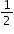of his portion of the pizza, what fraction of the whole pizza does he eat?

• Difficulty: N/A
• Type: EE: Equation Editor

• Test Item #: Sample Item 2
• Question:

In Homestead,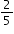of the households own pets. Of the households with pets,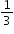have cats.

What fraction of the households in Homestead own cats?

• Difficulty: N/A
• Type: EE: Equation Editor

• Test Item #: Sample Item 3
• Question:

Sandy uses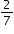of a pound of raisins in each batch of raisin bread.

Yesterday, Sandy used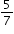of a pound of raisins. How many batches of raisin bread did sandy make yesterday?

• Difficulty: N/A
• Type: EE: Equation Editor

• Test Item #: Sample Item 4
• Question: Springfield has an elevation of −150 feet. Greenville is 3 times as far below sea level as Springfield.

What is Greenville’s elevation, in feet?

• Difficulty: N/A
• Type: EE: Equation Editor

• Test Item #: Sample Item 5
• Question:

An equation is shown, where z < 0.

x · y = z

A. Assume x > 0. Drag the point to the number line to identify a possible location for y.

B. Assume x < 0. Drag the point to the number line to identify a possible location for y.

• Difficulty: N/A
• Type: GRID: Graphic Response Item Display

• Test Item #: Sample Item 6
• Question:

What is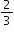written as a decimal?

• Difficulty: N/A
• Type: MC: Multiple Choice

## Related Courses

This benchmark is part of these courses.
1205020: M/J Accelerated Mathematics Grade 6 (Specifically in versions: 2014 - 2015, 2015 - 2020, 2020 - 2022, 2022 and beyond (current))
1205040: M/J Grade 7 Mathematics (Specifically in versions: 2014 - 2015, 2015 - 2022, 2022 and beyond (current))
1204000: M/J Foundational Skills in Mathematics 6-8 (Specifically in versions: 2014 - 2015, 2015 - 2022, 2022 and beyond (current))
1200410: Mathematics for College Success (Specifically in versions: 2014 - 2015, 2015 - 2022 (course terminated))
1200700: Mathematics for College Algebra (Specifically in versions: 2014 - 2015, 2015 - 2022 (course terminated))
7812020: Access M/J Grade 7 Mathematics (Specifically in versions: 2014 - 2015, 2015 - 2018, 2018 - 2019, 2019 - 2022, 2022 and beyond (current))
7912115: Fundamental Explorations in Mathematics 2 (Specifically in versions: 2013 - 2015, 2015 - 2017 (course terminated))

## Related Access Points

Alternate version of this benchmark for students with significant cognitive disabilities.

## Related Resources

Vetted resources educators can use to teach the concepts and skills in this benchmark.

## Educational Game

Integers Jeopardy Game:

This interactive game has 4 categories: adding integers, subtracting integers, multiplying integers, and dividing integers. Students can play individually or in teams.

Type: Educational Game

## Formative Assessments

Find Decimal Using Long Division:

Students are asked to use long division to convert four different fractions to equivalent decimals and to identify those that are rational.

Type: Formative Assessment

Understanding Products:

Students are asked to explain why the product of a positive and a negative rational number is negative.

Type: Formative Assessment

Negatives Explained:

Students are asked to describe a real-world context for a given expression involving the product of two rational numbers.

Type: Formative Assessment

Negative Times:

Students are shown a problem that illustrates why the product of two negatives is a positive and are asked to provide a rationale.

Type: Formative Assessment

Applying Rational Number Properties:

Students are asked to evaluate expressions involving multiplication of rational numbers and use the properties of operations to simplify calculations.

Type: Formative Assessment

Integer Division:

Students are asked to describe a real-world context for a given expression involving the quotient of two rational integers.

Type: Formative Assessment

Quotients of Integers:

Students are given an integer division problem and asked to identify fractions which are equivalent to the division problem.

Type: Formative Assessment

## Lesson Plans

Radioactive Dating Lesson 4 - Recursive Division :

This lesson introduces students to the idea of recursive division and its application to radioactive dating with a worksheet and Scratch programming. This is the final lesson in the Radioactive Dating Unit.

Type: Lesson Plan

Independent Compound Probability:

During this lesson, students will use Punnett Squares to determine the probability of an offspring's characteristics.

Type: Lesson Plan

Increasing and Decreasing Quantities by a Percent:

This lesson unit is intended to help you assess how well students are able to interpret percent increase and decrease, and in particular, to identify and help students who have the following difficulties:

• translating between percents
• decimals and fractions
• representing percent increase and decrease as multiplication
• recognizing the relationship between increases and decreases

Type: Lesson Plan

Let's Understand Multiplication of Positive and Negative Numbers:

This lesson provides teachers with a way to show students why a negative times a negative equals a positive. The lesson starts with a review of decomposition, the distributive property, and finding missing addends. Then with teacher guidance, groups of students apply these skills in a systematic way to apply properties of operations to discover the rules governing the signs of products for positive and negative factors and to multiply positive and negative numbers in mathematical and real-world problems. Finally, students independently show demonstrate mastery of the lesson objectives by completing an independent practice assessment.

Type: Lesson Plan

Increasing and Decreasing Quantities by a Percent:

This lesson unit is intended to help you assess how well students are able to interpret percent increase and decrease, and in particular, to identify and help students who have the following difficulties:

• Translating between percents, decimals, and fractions.
• Representing percent increase and decrease as multiplication.
• Recognizing the relationship between increases and decreases.

Type: Lesson Plan

Predicting the decimal equivalent for a fraction - terminating or repeating?:

This lesson encourages students to make an important discovery. Will a given fraction yield a terminating or repeating decimal? Discussion includes why knowing this is important. The lesson is structured to allow exploration, discovery, and summarization.

Type: Lesson Plan

## Original Student Tutorial

Why Does a Negative Times a Negative Equal a Positive?:

Use mathematical properties to explain why a negative factor times a negative factor equals a positive product instead of just quoting a rule with this interactive tutorial.

Type: Original Student Tutorial

Equivalent fractions approach to non-repeating decimals:

The purpose of the task is to get students to reflect on the definition of decimals as fractions (or sums of fractions), at a time when they are seeing them primarily as an extension of the base-ten number system and may have lost contact with the basic fraction meaning. Students also have their understanding of equivalent fractions and factors reinforced.

Repeating Decimal as Approximation:

The student is asked to complete a long division which results in a repeating decimal, and then use multiplication to "check" their answer. The purpose of the task is to have students reflect on the meaning of repeating decimal representation through approximation.

## Tutorials

Thinking About the Sign of Expressions:

This video shows some examples that test your understanding of what happens when positive and negative numbers are multiplied and divided.

Type: Tutorial

Converting repeating decimals to fractions :

Students will learn how to convert difficult repeating decimals to fractions.

Type: Tutorial

Converting repeating decimals to fractions :

This tutorial shows students how to convert basic repeating decimals to fractions.

Type: Tutorial

Converting a fraction to a repeating decimal:

Students will learn how to convert a fraction into a repeating decimal. Students should know how to use long division before starting this tutorial.

Type: Tutorial

Changing a Fraction to Decimal Form:

In this video, you will practice changing a fraction into decimal form.

Type: Tutorial

Multiplying and Dividing Even and Odd Numbers of Negatives:

You will learn how multiplication and division problems give us a positive or negative answer depending on whether there are an even or odd number of negative integers used in the problem.

Type: Tutorial

Simplifying Expressions with Rational Numbers:

In this tutorial, you will simplify expressions involving positive and negative fractions.

Type: Tutorial

Making Sense of Complex Fractions:

In this tutorial, you will see how to simplify complex fractions.

Type: Tutorial

Negative Signs in Numerators and Denominators:

In this tutorial, you will evaluate fractions involving negative numbers and variables to determine if expressions are equivalent.

Type: Tutorial

Dividing Negative Fractions:

In this tutorial, you will see how to divide fractions involving negative integers.

Type: Tutorial

Multiplying Negative and Positive Fractions:

In this tutorial you will practice multiplying and dividing fractions involving negative numbers.

Type: Tutorial

Multiplying Positive and Negative Numbers:

In this tutorial, you will learn rules for multiplying positive and negative integers.

Type: Tutorial

Dividing Positive and Negative Numbers:

In this tutorial you will learn how to divide with negative integers.

Type: Tutorial

Why a Negative Times a Negative Makes a Positive:

In this tutorial you will use the repeated addition model of multiplication to help you understand why multiplying negative numbers results in a positive answer.

Type: Tutorial

Why a Negative Times a Negative is a Positive:

In this tutorial, you will use the distributive property to understand why the product of two negative numbers is positive.

Type: Tutorial

Pre-Algebra - Fractions and Rational Numbers:

The first fractions used by ancient civilizations were "unit fractions." Later, numerators other than one were added, creating "vulgar fractions" which became our modern fractions. Together, fractions and integers form the "rational numbers."

Type: Tutorial

Pre-Algebra - Multiplying Negative Numbers:

When number systems were expanded to include negative numbers, rules had to be formulated so that multiplication would be consistent regardless of the sign of the operands.

Type: Tutorial

Multiplying Fractions:

The video describes how to multiply fractions and state the answer in lowest terms.

Type: Tutorial

## Video/Audio/Animation

Converting Fractions to Decimal Numbers:

Any fraction can be converted into an equivalent decimal number with a sequence of digits after the decimal point, which either repeats or terminates. The reason can be understood by close examination of the number line.

Type: Video/Audio/Animation

## MFAS Formative Assessments

Applying Rational Number Properties:

Students are asked to evaluate expressions involving multiplication of rational numbers and use the properties of operations to simplify calculations.

Find Decimal Using Long Division:

Students are asked to use long division to convert four different fractions to equivalent decimals and to identify those that are rational.

Integer Division:

Students are asked to describe a real-world context for a given expression involving the quotient of two rational integers.

Negative Times:

Students are shown a problem that illustrates why the product of two negatives is a positive and are asked to provide a rationale.

Negatives Explained:

Students are asked to describe a real-world context for a given expression involving the product of two rational numbers.

Quotients of Integers:

Students are given an integer division problem and asked to identify fractions which are equivalent to the division problem.

Understanding Products:

Students are asked to explain why the product of a positive and a negative rational number is negative.

## Original Student Tutorials Mathematics - Grades 6-8

Why Does a Negative Times a Negative Equal a Positive?:

Use mathematical properties to explain why a negative factor times a negative factor equals a positive product instead of just quoting a rule with this interactive tutorial.

## Student Resources

Vetted resources students can use to learn the concepts and skills in this benchmark.

## Original Student Tutorial

Why Does a Negative Times a Negative Equal a Positive?:

Use mathematical properties to explain why a negative factor times a negative factor equals a positive product instead of just quoting a rule with this interactive tutorial.

Type: Original Student Tutorial

## Educational Game

Integers Jeopardy Game:

This interactive game has 4 categories: adding integers, subtracting integers, multiplying integers, and dividing integers. Students can play individually or in teams.

Type: Educational Game

Repeating Decimal as Approximation:

The student is asked to complete a long division which results in a repeating decimal, and then use multiplication to "check" their answer. The purpose of the task is to have students reflect on the meaning of repeating decimal representation through approximation.

## Tutorials

Thinking About the Sign of Expressions:

This video shows some examples that test your understanding of what happens when positive and negative numbers are multiplied and divided.

Type: Tutorial

Converting repeating decimals to fractions :

Students will learn how to convert difficult repeating decimals to fractions.

Type: Tutorial

Converting repeating decimals to fractions :

This tutorial shows students how to convert basic repeating decimals to fractions.

Type: Tutorial

Converting a fraction to a repeating decimal:

Students will learn how to convert a fraction into a repeating decimal. Students should know how to use long division before starting this tutorial.

Type: Tutorial

Changing a Fraction to Decimal Form:

In this video, you will practice changing a fraction into decimal form.

Type: Tutorial

Multiplying and Dividing Even and Odd Numbers of Negatives:

You will learn how multiplication and division problems give us a positive or negative answer depending on whether there are an even or odd number of negative integers used in the problem.

Type: Tutorial

Simplifying Expressions with Rational Numbers:

In this tutorial, you will simplify expressions involving positive and negative fractions.

Type: Tutorial

Making Sense of Complex Fractions:

In this tutorial, you will see how to simplify complex fractions.

Type: Tutorial

Negative Signs in Numerators and Denominators:

In this tutorial, you will evaluate fractions involving negative numbers and variables to determine if expressions are equivalent.

Type: Tutorial

Dividing Negative Fractions:

In this tutorial, you will see how to divide fractions involving negative integers.

Type: Tutorial

Multiplying Negative and Positive Fractions:

In this tutorial you will practice multiplying and dividing fractions involving negative numbers.

Type: Tutorial

Multiplying Positive and Negative Numbers:

In this tutorial, you will learn rules for multiplying positive and negative integers.

Type: Tutorial

Dividing Positive and Negative Numbers:

In this tutorial you will learn how to divide with negative integers.

Type: Tutorial

Why a Negative Times a Negative Makes a Positive:

In this tutorial you will use the repeated addition model of multiplication to help you understand why multiplying negative numbers results in a positive answer.

Type: Tutorial

Why a Negative Times a Negative is a Positive:

In this tutorial, you will use the distributive property to understand why the product of two negative numbers is positive.

Type: Tutorial

Pre-Algebra - Fractions and Rational Numbers:

The first fractions used by ancient civilizations were "unit fractions." Later, numerators other than one were added, creating "vulgar fractions" which became our modern fractions. Together, fractions and integers form the "rational numbers."

Type: Tutorial

Pre-Algebra - Multiplying Negative Numbers:

When number systems were expanded to include negative numbers, rules had to be formulated so that multiplication would be consistent regardless of the sign of the operands.

Type: Tutorial

Multiplying Fractions:

The video describes how to multiply fractions and state the answer in lowest terms.

Type: Tutorial

## Video/Audio/Animation

Converting Fractions to Decimal Numbers:

Any fraction can be converted into an equivalent decimal number with a sequence of digits after the decimal point, which either repeats or terminates. The reason can be understood by close examination of the number line.

Type: Video/Audio/Animation

## Parent Resources

Vetted resources caregivers can use to help students learn the concepts and skills in this benchmark.

Repeating Decimal as Approximation:

The student is asked to complete a long division which results in a repeating decimal, and then use multiplication to "check" their answer. The purpose of the task is to have students reflect on the meaning of repeating decimal representation through approximation.